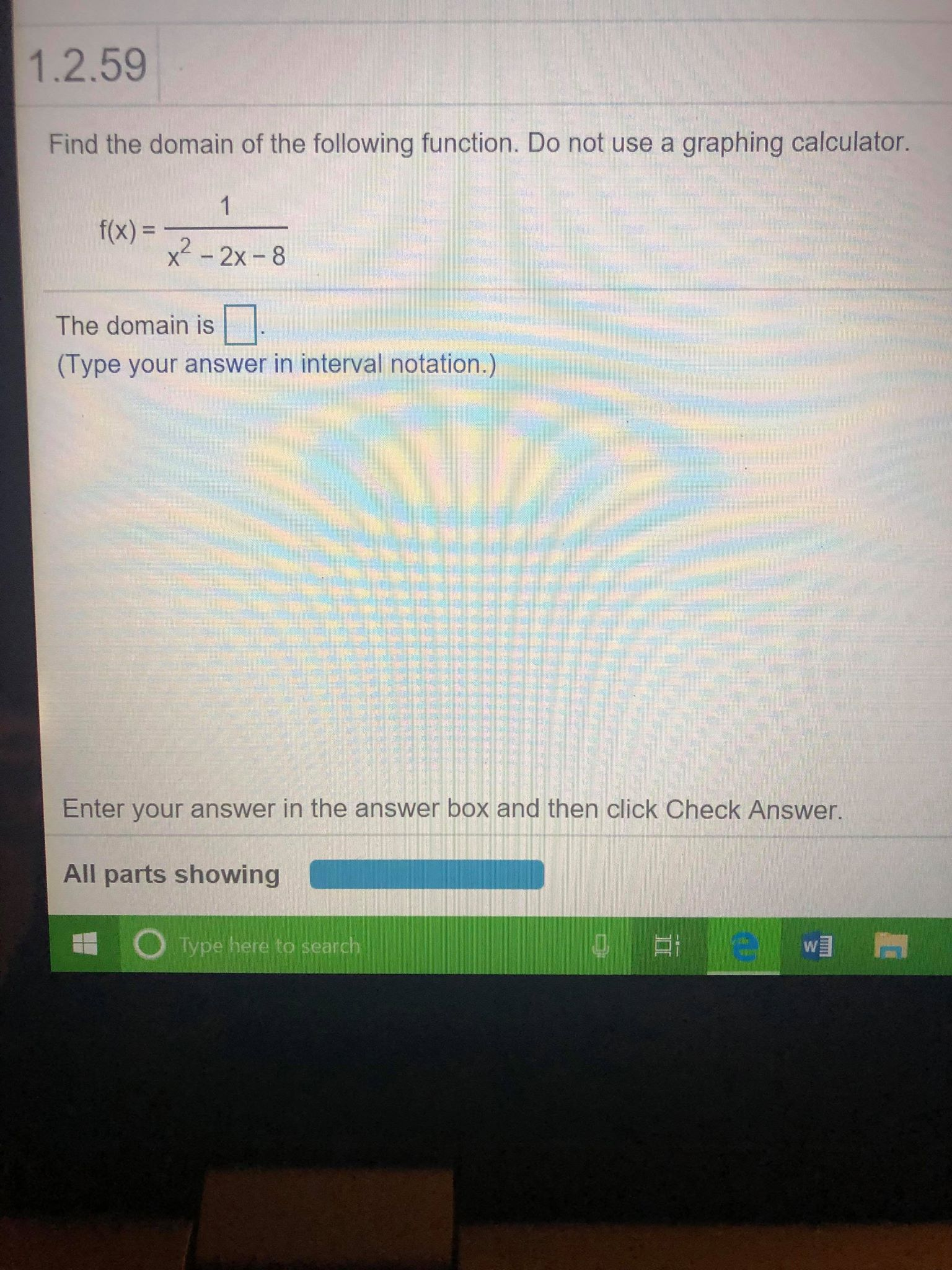# 1.2.59 Find the domain of the following function. Do not use a graphing calculator. f(x) = x2 -2x-8 The domain is □ (Type your answer in interval notation.) Enter your answer in the answer box and then click Check Answer. All parts showing Type here to search

Questionhelp_outlineImage Transcriptionclose1.2.59 Find the domain of the following function. Do not use a graphing calculator. f(x) = x2 -2x-8 The domain is □ (Type your answer in interval notation.) Enter your answer in the answer box and then click Check Answer. All parts showing Type here to search fullscreen